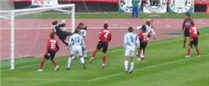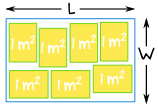# 解不等式应用题

（你也许想先去看不等式入门解不等式。）## 把语文转换为代数

• 先把问题从头到尾看一次
• 如有需要，画个草图
• 英语字母代替每个值
• 找出合适的公式### 小山和小李在同一个足球队踢球。 上星期六小李比小山多进了3球，但他们两个人进的球总共少于9球。 小李可能进了多少球？

• 小李进的球为：A
• 小山进的球为：S

#### 解：

 开始： S + A < 9 A = S + 3，所以可以 把 "S + 3" 代入 A： S + (S + 3) < 9 简化： 2S + 3 < 9 每边减 3： 2S < 9 − 3 简化： 2S < 6 每边除以 2： S < 3

• 当 S = 0，则 A = 3， S + A = 3，3 < 9 是对的
• 当 S = 1，则 A = 4， S + A = 5，5 < 9 是对的
• 当 S = 2，则 A = 5， S + A = 7，7 < 9 是对的
• （但当 S = 3，则 A = 6， S + A = 9，9 < 9 是错的）

## 很多例子！### 例子：8条小狗里，雌的比雄的要多。 可能有几条雌性小狗？

• 雌性小狗的数目：g
• 雄性小狗的数目：b

b = 8 − g

g > b

 开始： g > b b = 8 − g，所以我们可以 把 "8 − g" 代入 b: g > 8 − g 每边加 g： g + g > 8 简化： 2g > 8 每边除以 2： g > 4

• 当 g = 8，则 b = 0， g > b 是对的（可是，允许 b = 0 吗？）
• 当 g = 7，则 b = 1， g > b 是对的
• 当 g = 6，则 b = 2， g > b 是对的
• 当 g = 5，则 b = 3， g > b 是对的
• （但若 g = 4，则 b = 4，g > b 便是错的）### 例子：祖尔参加了一个需要骑自行车和跑的竞赛 他骑了 25千米的自行车，然后跑了 20千米。他跑的平均速度是骑自行车的平均速度的一半。 祖尔用了少于 2½ 小时完成比赛，他的平均速度是多少？

• 平均跑速： s
• 所以骑自行车的平均速度：2s

• 速度 = 距离 / 时间
• 重排为：时间 = 距离 / 速度

#### 1. 骑自行车

• 距离 = 25 km
• 平均速度 = 2s km/h
• 故此， 时间 = 距离/平均速度 = 25/2s 小时

#### 2. 跑

• 距离 = 20 km
• 平均速度 = s km/h
• 故此， 时间 = 距离/平均速度 = 20/s 小时

• 总时间 < 2½
• 25/2s + 20/s < 2½

 开始： 25/2s + 20/s < 2½ 把所有项乘以 2s： 25 + 40 < 5s 简化： 65 < 5s 每边除以 5： 13 < s 换边： s > 13### 例子：把球垂直向上抛，球的速度，v m/s，的公式是 v = 20 – 10t，其中 t 是时间（秒）。 在什么时间球的速度是在 10 m/s 和 15 m/s 之间？

• 速度（m/s）：v
• 时间（秒）：t

• v = 20 – 10t

10  <  v  <  15
10  <  20 − 10t  <  15

 开始： 10  <  20 − 10t  <  15 全部减 20： 10 − 20  <  20 − 10t − 20  <  15 − 20 简化： −10  < −10t  <  −5 除以 10： −1  < −t  <  −0.5 改变正负号，同时倒转不等号： 1  >  t  >  0.5 把较小的数放在前面比较整齐，所以： 倒过来： 0.5  <  t  <  1

### 例子：一个长方形的房间可以放 7 张面积 1 平方米的桌子，房间的周长是 16米。 房间的长度和宽度可能是多少？• 房间的长度：L
• 房间的宽度：W

• 2(W + L) = 16
• W + L = 8
• L = 8 − W

• W × L ≥ 7

 开始： W × L ≥ 7 代入 L = 8 − W: W × (8 − W) ≥ 7 展开： 8W − W2 ≥ 7 把所有的项移到左边： W2 − 8W + 7 ≤ 0

 把数字项 −7 移到右边： W2 − 8W ≤ −7 左边配方，把相同的值加到右边以保持平衡： W2 − 8W + 16 ≤ −7 + 16 简化： (W − 4)2 ≤ 9 每边取平方根： −3 ≤ W − 4 ≤ 3 对了，有两个不等式，因为 32 = 9，而同时 −32 = 9 每边加 4： 1 ≤ W ≤ 7

• 若 W = 1，则 L = 8−1 = 7，A = 1 x 7 = 7 m2 （刚好放下 7张桌子）
• 若 W = 0.9 （小于 1），则 L = 7.1， A = 0.9 x 7.1 = 6.39 m2 （放不进 7张桌子）
• 若 W = 1.1 （刚大于 ，则 L = 6.9， A = 1.1 x 6.9 = 7.59 m2 （很容易放进 7张桌子）
• W 在 7 m 附近也一样